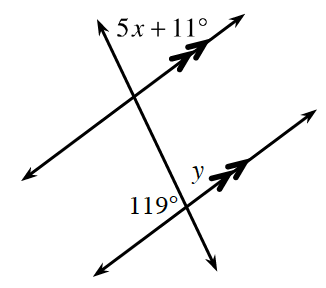### Home > GC > Chapter 3 > Lesson 3.1.2 > Problem3-20

3-20.Examine the relationships in the diagram at right. Then solve for $x$ and $y$, if possible.

$∠119º$ and $∠y$ are supplementary angles, and $∠y$ and $∠5x+11º$ are corresponding angles.

See the homework help for problem 2-55 in Lesson 2.1.5 if you need further help solving for $x$ and $y$.